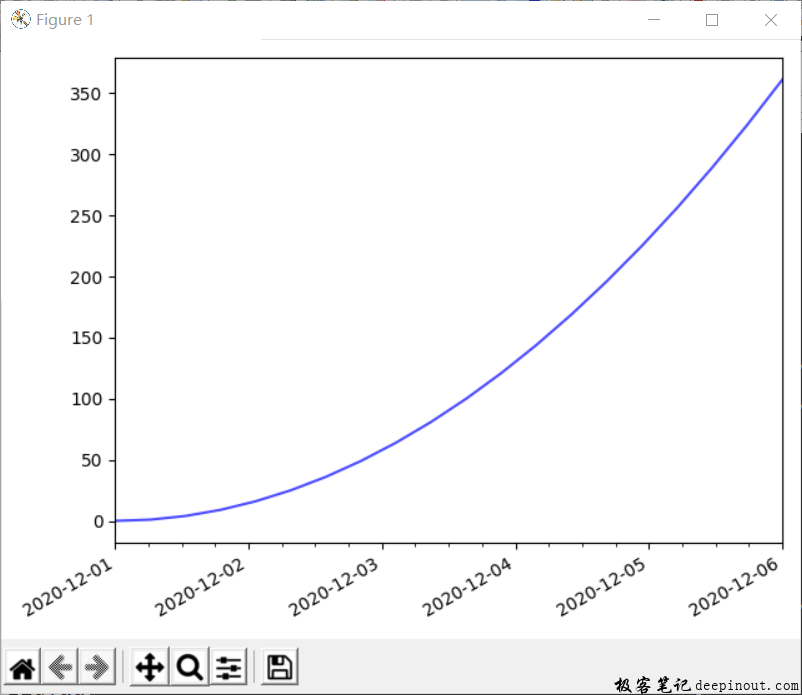# Matplotlib X轴年份采用四位显示host.xaxis.set_major_formatter(mdates.DateFormatter('%Y-%m-%d'))

host.xaxis.set_minor_locator(mdates.HourLocator(range(0, 25, 6)))

host.tick_params(axis = "both", direction = "out", labelsize = 10)

date1 = datetime.date(2020, 12, 1)

date2 = datetime.date(2020, 12, 6)

delta = datetime.timedelta(hours=6)

dates = mdates.drange(date1, date2, delta)

print(dates)


host.set_xlim(dates, dates[-1])

y = np.arange(len(dates))

host.plot_date(dates, y**2, 'b-', alpha = 0.7)


import numpy as np
import matplotlib.pyplot as plt
import matplotlib.dates as mdates
import datetime

#
fig, host = plt.subplots()  #创建子图
host.grid(False)

#
host.xaxis.set_major_formatter(mdates.DateFormatter('%Y-%m-%d'))
host.xaxis.set_minor_locator(mdates.HourLocator(range(0, 25, 6)))
host.tick_params(axis = "both", direction = "out", labelsize = 10)

date1 = datetime.date(2020, 12, 1)
date2 = datetime.date(2020, 12, 6)
delta = datetime.timedelta(hours=6)
dates = mdates.drange(date1, date2, delta)
print(dates)
host.set_xlim(dates, dates[-1])

y = np.arange(len(dates))
host.plot_date(dates, y**2, 'b-', alpha = 0.7)

fig.autofmt_xdate()

plt.tight_layout()
plt.show()


• 回顶